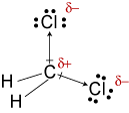# What kind of intermolecular forces are present in the following compounds: C Cl_4, CH_2Cl_2, CH_3OH, CO_2, SCl_4, and SCl_6?

Aug 16, 2016

Warning! Long answer. Here's what I get.

You have to draw the Lewis structure of each molecule, use VSEPR theory to determine its shape, and then decide whether or not the bond dipoles cancel.

${\text{CO}}_{2}$ and ${\text{CCl}}_{4}$(From www.peoi.org)

${\text{CO}}_{2}$ is a linear molecule with an $\text{O-C-O}$ bond angle of 180°. The bond dipoles are equal and in opposite directions, so they cancel.

${\text{CO}}_{2}$ is a nonpolar molecule. Its strongest intermolecular forces are London dispersion forces.

${\text{CCl}}_{4}$ is a tetrahedral molecule with a $\text{Cl-C-Cl}$ bond angle of 109.5°.

The two $\text{C-Cl}$ bond dipoles in the plane of the paper have a resultant pointing to the right at an angle of 54.75° from the vertical.

The two $\text{C-Cl}$ bond dipoles behind and in front of the paper have an equal and opposite resultant to the first.

Since the bond dipoles are equal and in opposite directions, they cancel.

${\text{CCl}}_{4}$ is a nonpolar molecule. Its strongest intermolecular forces are London dispersion forces.

${\text{CH"_2"Cl}}_{2}$${\text{CH"_2"Cl}}_{2}$ has a tetrahedral shape. The two $\text{C-Cl}$ bond dipoles have a resultant that bisects the $\text{Cl-C-Cl}$ bond angle.

${\text{CH"_2"Cl}}_{2}$ is therefore a polar molecule, and its strongest intermolecular forces are dipole-dipole forces.

$\text{CH"_3"OH}$

$\text{CH"_3"OH}$ has a highly polar $\text{O-H}$ bond. The $\text{O}$ atom has a high δ^"-" charge, and the $\text{H}$ of the $\text{OH}$ has a high δ^+ charge.The $\text{O}$ in one molecule is strongly attracted to the $\text{H}$ in another molecule, and the $\text{H}$ in one molecule is strongly attracted to the $\text{O}$ in another molecule.

The strongest intermolecular force in $\text{CH"_3"OH}$ is hydrogen bonding.

${\text{SCl}}_{4}$(From en.wikipedia.org)

${\text{SCl}}_{4}$ has a see-saw shape.

The two horizontal $\text{S-Cl}$ bond dipoles cancel, but the downward-pointing dipoles reinforce each other.

${\text{SCl}}_{4}$ is a polar molecule, and its strongest intermolecular forces are dipole-dipole forces.

${\text{SCl}}_{6}$

${\text{SCl}}_{6}$is an octahedral molecule.Every $\text{S-Cl}$ bond dipole has a partner pointing in exactly the opposite direction, so all bond dipoles cancel.

${\text{SCl}}_{6}$ is a nonpolar molecule, so its strongest intermolecular forces are London dispersion forces.